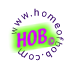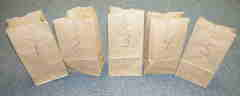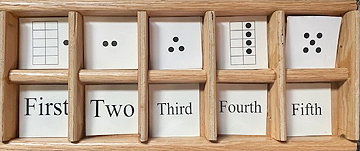# Numbers: values and basic operations

## Knowledge base & tools to develop mathematical literacy

### Knowledge base

Mathematical knowledge base with concepts necessary for learners to conceptualize to become mathematically literate and misconceptions that must be over come. Particularly important for how learners develop their understanding of numbers, their values, operations, patterns, and relationships are:

Concepts related to:

## Information to facilitate literacy in numbers (values & basic operations) & tools to achieve it

Numbers & their definitions - Fact sheet of natural, integer, rational, irrational and real numbers. Their definitions, examples, & number line visual representation.

### Numbers: Counting, recognition, cardinality

Related activities

### Place value development & assessment

Development

• Place value- development from age 5 on: ten as big number, group by ten, pre place value, unitize, place value relationships, decimals, exponents, place value & decimal worksheets

Assessment

### Fractions, ratio, & proportion development & assessment

Development

Related activities

### Operations

#### Addition & subtraction - whole number development & assessment

Development

Related activities

Assessment

• Addition and Subtraction - suggestions, directions with problems, scoring guides, & score sheets to assess the development of + & - from four ways: join, separate, part-part-whole, & combine with scoring guide ideas to understanding and use of algorithms

#### Multiplication & division of whole number development & assessment

Development

Related activities

#### Addition, subtraction, multiplication, & division - fractional numbers development & assessment

Division problems represented with squares and rectangles

• Sample problems: 1/2 ÷ 1/2, 1/2 ÷ 1/4 , 1/2 ÷ 3/4, 1/2 ÷ 3/5 (thanks Brant),

### Number sense - whole numbers

Number relationships --->>> see patterns and algebra

#### Manipulatives for students to use to learn number valueCards for learners to sort - make a set of cards by combining cards appropriate for a particular learner: numerals, words, ordinal, dot, ten frame with dots. The cards can be sorted into paper bags, a sorting box, or on any flat surface. Different games or activities can be created: sort dots, sort dots and label with the set's cardinality numeral word, sort and label with ordinal word, sort all cards and beat the clock ...

Sample cards to print and cut out:Dice game - helps students subitize number value and introduction to addition. Roll the dice and flip the values on the die or the sum of the die. Continue to roll until all the numbers (1-12) are flipped (win) or a value rolled has had all it's possible plays, already flipped. Example: roll 2 & 2. Can flip 4, or 1 & 3.

Die roll - simulate a roll of a die.

Number line: is a good mathematical tool to represent mathematical values and operations. However, children who are not familiar with them and confident with their use, are not sure what to count: numbers, lines, spaces and where to start (0, 1, ...).

Therefore, before they’re used as a representation to explain and prove mathematical ideas, learners need experiences to develop their understanding and fluency with their use.

Activities, used when counting, such as numbered polka dots organized in a line can provide good background before introducing a number line.### Really big numbers and infinity

• Olber's Paradox - if the universe is infinite in time and space, stars should occupy every point in space and fill the night sky with light.
• How many grains of sand in the Universe? Archimedes' proof in The Sand Reckoner.

### Number Theory

Number theory is critical for students to do well in algebra and higher levels of mathematics. However, the sad thing is most teachers are unfamiliar with these ideas, or skip them when they occur in their math text or program, as their view of mathematics is limited to basic facts and operations as calculations of numbers.

The following are examples related to number theory.

### Basic Operations (+-*/)

#### Fractional numbers (+ - * /)

Activities for number sense particularly: fractions, decimals, and percents

Division problems represented with squares and rectangles

• Sample problems: 1/2 ÷ 1/2, 1/2 ÷ 1/4 , 1/2 ÷ 3/4, 1/2 ÷ 3/5 (thanks Brant),

#### Decimal numbers (+ - * /)

Activities for number sense particularly: fractions, decimals, and percents

### Integer values and operations (+ - * /)

Addition and subtraction of positive and negative integers might be conceptualized by representing and controling two properties: position on a number line and direction of body.

The number line can have two halves with the same numbers being both positive and negative. You have two choices for each number + or - as a reference to position. If the number has a value of 4, it could be represented as a positive four or negative four.

You can represent those two numbers by standing on either +4 or -4. Secondly when you are standing on a number line you can face one of two direction, since the number line is bidirectional, you might be facing left or right, forward or backward, in a positive direction or negative direction. or if it is thought of as a thermometer, you can walk hotter or colder.

Problems can be posed as:
Find a starting point, say -3 and if you add a +3, face warmer and walk forward 3.
Find a starting point, say -3 and if + -3, then face warmer and walk backward.
Find a starting point, say -3 and if - - 3, then face colder and walk backward.
Find a starting point, say -3 and if - +3, then face colder and walk forward.

Again both are important and a concrete model can be used to describe a procedural rule, as demonstrated as a plus and a plus are plus, a plus and a minus are a minus, and a minus and a minus are a plus.

Another idea is to record a video with motion. People walking or running forward and backward. They might display signs with forward and backward as they do so. However, it probably will be obvious which direction they moving when it is recorded. When the video is made, then each can be viewed with the movie play forward and backward. A chart of the different results can be made (forward, forward, results in forward; forward, backward, results in backward; backward, forward results in backward; backward, backward results in forward.

After several concrete experience have been experienced explore the this challenge.
How many different ways can two numbers be represented with different a sign and an operation (-2 ++3; -2 -+3; -2 --3; -2 +-3) (2 ++3; 2 -+3; 2 --3; 2 +-3).

Other development & activities pages:

Process dimensions:

Content dimensions:

If the universe is infinite in time and space, stars should occupy every point in space and fill the night sky with light.